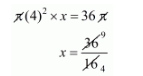# A cylindrical vessel of radius 4 cm contains water.`
Question:

A cylindrical vessel of radius 4 cm contains water. A solid sphere of radius 3 cm is lowered into the water until it is completely immersed. The water level in the vessel will rise by

(a) $\frac{2}{9} \mathrm{~cm}$

(b) $\frac{4}{9} \mathrm{~cm}$

(c) $\frac{9}{4} \mathrm{~cm}$

(d) $\frac{9}{2} \mathrm{~cm}$

Solution:

The radius of sphere, r = 3 cm

The volume of sphere

$=\frac{4}{3} \pi r^{3}$

$=\frac{4}{3} \pi(3)^{3}$

$=36 \pi \mathrm{cm}^{3}$

Since,

The sphere fully immersed into the vessel, the level of water be raised by x cm.

Then,

The volume of raised water = volume of sphere$x=\frac{9}{4} \mathrm{~cm}$

Hence, the correct answer is choice (c).